# CBEST Math Practice Test

CBEST Math Test Prep

If you are serious about getting a great score on your CBEST Math test, try out our recommended CBEST Math Prep Course.

Use our free CBEST Math practice test to review the most important skills and concepts that you must know for this certification exam. Our CBEST math test includes 50 multiple choice practice questions with detailed explanations. You are not allowed to use a calculator.

Congratulations - you have completed .

You scored %%SCORE%% out of %%TOTAL%%.

Your performance has been rated as %%RATING%%

 Question 1

### Which of the following is the BEST estimate of 3995 × 102?

 A 450,000 B 400,000 C 350,000 D 300,000 E 250,000
Question 1 Explanation:
The correct answer is (B). The number 3995 is close to 4000, and the number 102 is close to 100. The estimated product can then be written as (4000)(100) = 400,000. (Note that the actual product is 407,490.)
 Question 2

### Gina buys nine pens at \$1.10 per pen. Which of the following represents the change she would receive if she purchased the pens using a \$20 bill?

 A \$20 – (9)(\$1.10) B (\$20 – 9)(\$1.10) C \$20 + (9)(\$1.10) D (\$20 – \$1.10)(9) E \$20 – 9 – \$1.10
Question 2 Explanation:

### Which of the following methods could Jake also use to determine the sale price?

 A $\frac{25}{200}$ × 70 B 70 ÷ 0.25 C (1 − 0.25) × 70 D 70 − 25 E 70 ÷ (1 + 0.25)
Question 25 Explanation:
The correct answer is (C). Let's start by looking the expression that is given in the question:

70 − (0.25 × 70)

Here we are taking the original amount and subtracting away 25% of the original amount.

If we take away 25% of the original amount, we will be left with 75% of the original amount.

0.75 × 70 would give the same result.

We can rewrite this as:

(1 − 0.25) × 70
 Question 26

### Jon likes to tip 20% at restaurants. To figure out the tip he should leave for a \$35 meal, he uses the following expression: ###$0.20 × 35$### Which of the following expressions could Jon have also used?  A$\dfrac{35}{10} × 2$B$35 ÷ (1 − 0.20) − 35$C$\dfrac{35 × 100}{20}$D$\dfrac{35 − 20}{2}$E$35 ÷ 4$Question 26 Explanation: The correct answer is (A). Dividing any number by 10 will give you the value of 10% of the original number. So,$\frac{35}{10}$gives us 3.50 which is 10% of 35. To find 20% of 35, we simply double the value we found for 10%. Thus,$\frac{35}{10}$× 2 = 7.00 provides the correct result.  Question 27 ### If a bus travels 360 kilometers in 5 hours, how many kilometers will it travel in 9 hours when driving at the same speed?  A 72 B 288 C 620 D 648 E 660 Question 27 Explanation: The correct answer is (D). First calculate the distance the bus travels in 1 hour: 360 km ÷ 5 hours = 72 km per hour Then multiply this number by the total number of hours traveled: 9 hours × 72 km per hour = 648 km Notice that the final calculation yields the correct units; this helps to confirm that the calculation is correct.  Question 28 ### A vase contains 60 marbles, all of which are red or orange. If the ratio of red marbles to orange ones is 1:5, what is the total number of red marbles in the vase?  A 8 B 10 C 12 D 15 E 50 Question 28 Explanation: The correct answer is (B). We know that the ratio is 1:5. This means that for every six marbles one of them is red and five are orange. So$\frac{1}{6}$of the marbles are red:$\frac{1}{6}$× 60 = 10  Question 29 ### Cameron purchased a new hat that was on sale for \$5.40. The original price was \$9.00. What percentage discount was the sale price?  A 5.4% B 54.0% C 40.0% D 60.0% E 45.0% Question 29 Explanation: The correct answer is (C). The percentage discount is the reduction in price divided by the original price. The difference between original price and sale price is:$\$9.00 − \$5.40 = \$3.60$

The percentage discount is this difference divided by the original price:

$\$3.60 ÷ \$9.00 = \dfrac{3.60}{9.00}$

$= \dfrac{360}{900}$ $= \dfrac{40}{100}$ $= 40 \%$
 Question 30### Neil ordered the following for his family: two cheeseburgers, one hamburger, two large fries, one small fries, and three small sodas. If the total calories for the order is 3370, what is the missing calorie information on the menu?

 A 1000 B 400 C 450 D 480 E 900
Question 30 Explanation:
The correct answer is (C). Let's begin by totaling the calories for the items we are able to:

Two cheeseburgers = 2 × 530 = 1,060 calories

One hamburger = 430 calories

One small fries = 230 calories

Three small sodas = 3 × 250 = 750 calories

If we subtract each of the known calories from the total calories for the order we will know how many calories came from two large fries:

3,370 − 1,060 − 430 − 230 − 750 = 900

900 calories came from two orders of large fries. Each order of fries must be 450 calories.
 Question 31

### Use the table below to answer the question that follows.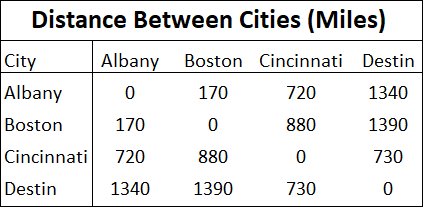### If a truck travels from Boston to Albany to Cincinnati to Destin, how many miles will the truck travel in total?

 A 1620 miles B 2440 miles C 1390 miles D 2230 miles E 1780 miles
Question 31 Explanation:
The correct answer is (A). Let's determine the distance traveled for each of the three legs of the trip using the provided table:

Boston to Albany = 170 miles

Albany to Cincinnati = 720 miles

Cincinnati to Destin = 730 miles

The total distance = 170 + 720 + 730 = 1620 miles
 Question 32

### Use the table below to answer the question that follows.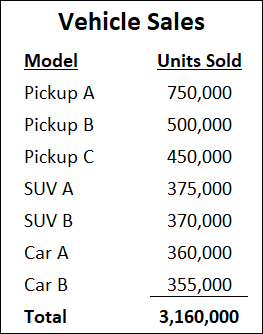### What percentage of the seven vehicles shown in the table were pickups?

 A 46% B 5% C 60% D 16% E 54%
Question 32 Explanation:
The correct answer is (E). To find the percentage of vehicles that were pickups, we need to first divide the total number of pickups sold by the total number of all seven vehicles sold:

$\frac{(750,000 + 500,000 + 450,000)}{3,160,000}$ $= \frac{1,700,000}{3,160,000} =$ 0.538

To convert to a percentage, we need to multiply by 100.

0.538 × 100 = 53.8%

 Question 33

### A restaurant cooked $3\frac{2}{5}$ pounds of rice before opening, and another $1\frac{4}{7}$ pounds later in the afternoon. What was the total amount that they cooked?

 A $\dfrac{145}{34} \text { pounds}$ B $\dfrac{174}{35} \text { pounds}$ C $\dfrac{168}{35} \text { pounds}$ D $\dfrac{159}{34} \text { pounds}$ E $\dfrac{119}{35} \text { pounds}$
Question 33 Explanation:
The correct answer is (B). To add mixed number fractions, first convert them to improper fractions, then find a common denominator and add the numerators, placing them over the common denominator. Reduce the final fraction if necessary.

To convert a mixed fraction to an improper fraction, multiply the whole number with the denominator, add this to the numerator, and place the resulting value above the original denominator.

$3\dfrac{2}{5} = \dfrac{17}{5}$

$1\dfrac{4}{7} = \dfrac{11}{7}$

Now find the lowest common denominator between 5 and 7. Since they are both prime numbers, their lowest common denominator is their product, 35. We can rewrite both fractions:

$\dfrac{17}{5} = \dfrac{119}{35}$

$\dfrac{11}{7} = \dfrac{55}{35}$

Now that the denominators are the same, we can add the numerators:

$\dfrac{119}{35} + \dfrac{55}{35} = \dfrac{119 + 55}{35}$ $= \dfrac{174}{35}$
 Question 34

### $w = 500q + 35{,}000$

 A The combined weight of the truck and a full load of castings B The weight of the truck with no load C The weight of each casting D The maximum weight of castings that the truck can haul E The total weight of the castings
Question 34 Explanation:
The correct answer is (B). We are told that $w$ represents the total weight of the truck and its load, and $q$ represents the quantity of castings it is hauling.

If a truck is hauling no castings, $q = 0$.

When $q = 0$, $w = 500(0) + 35,000$ $= 35,000$.

In other words, the weight of the truck by itself (i.e. hauling no castings) is 35,000 lbs.
 Question 35

### Kara buys groceries once a week. In the past four visits she has spent \$116.25, \$130.07, \$128.82, and \$109.53. If the individual amounts spent are rounded to the nearest \$10, what is the estimate of Kara's total spending for all four visits?  A$480 B $484.70 C$490 D $484.67 E$460
Question 35 Explanation:
The correct answer is (C). Begin by rounding each of the four amounts to the nearest \$10: \$116.25 → Closer to \$110 or \$120? → \$120 \$130.07 → Closer to \$130 or \$140? → \$130 \$128.82 → Closer to \$120 or \$130? → \$130 \$109.53 → Closer to \$100 or \$110? → \$110 To find total spending, we sum up our four estimates: \$120 + \$130 + \$130 + \$110 = \$490
 Question 36

### Aaron determines that 23 gallons of gas are used by his lawn care business in a typical week. If the gas usage is rounded to the nearest 5 gallons, how many gallons would he expect to use in 12 weeks?

 A 300 gallons B 320 gallons C 276 gallons D 240 gallons E 287 gallons
Question 36 Explanation:
The correct answer is (A). First, we need to round 23 gallons to the nearest 5 gallons:

23 → Closer to 20 or 25? → 25

Next, we need to multiply 25 by 12:

$\require{cancel} \dfrac{25 \text{ gallons}}{\cancel{\text{week}}} × 12 \require{cancel} \cancel{\text{weeks}}$ $= 300 \text{ gallons}$
 Question 37

### Use the information below to answer the question that follows.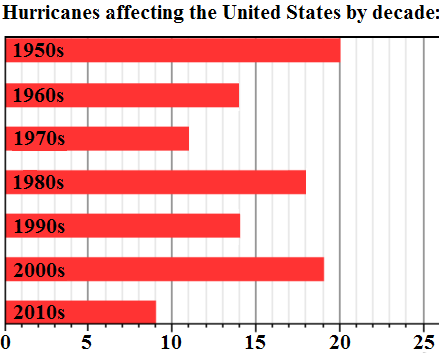### What is the average number of hurricanes affecting the US per decade?

 A 11 B 13 C 14 D 15 E 18
Question 37 Explanation:
The correct answer is (D). To find the average, add up all the numbers, then divide by how many numbers there are:

$\text{Avg} =$ $\frac{20 + 14 + 11 + 18 + 14 + 19 + 9}{7}$

$\text{Avg} = \frac{105}{7} = 15$
 Question 38

### Use the information below to answer the question that follows.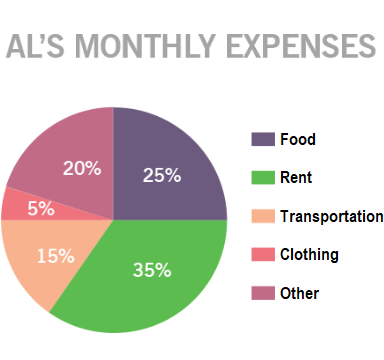### This pie chart shows Al’s monthly expenses. If Al spent a total of $1,550 in one month, how much did he spend on clothes in that month?  A$77.50 B $232.50 C$775 D $7.75 E$1,627.50
Question 38 Explanation:
The correct answer is (A). From the pie chart it can be seen that clothing represents 5% of his monthly expenses. Recall that 5% is equivalent to $\frac{5}{100}$ or 0.05:

0.05 × \$1,550 = \$77.50
 Question 39

### Which of the following expressions could Tina have also used?

 A 5 ÷ 2 B (6 × 2) ÷ 5 C $\frac{2}{5}$ × 6 D (6 ÷ 5) × 2 E $\frac{5}{2}$ × 6
Question 39 Explanation:
The correct answer is (E). Consider Tina's first expression, (6 × 5) ÷ 2. First, she determines the total amount of iced tea:

$6 \require{cancel} \cancel{\text{jugs}}$ × $\require{cancel} \dfrac{5 \text{ jugs}}{\cancel{\text{jug}}}$ $= 30 \text{ jugs}$

Next, she determines how many 2-gallon jugs would be required to hold the same amount:

$30 \require{cancel} \cancel{\text{gallons}} × \require{cancel} \dfrac{1 \text{ jug}}{ 2\cancel{\text{gallons}}}$ $= 15 \text{ jugs}$

An alternate approach would use the expression,

$\dfrac{5}{2} × 6$

First, she determines how many 2-gallon jugs are contained within each 5-gallon jug:

5 $\require{cancel} \cancel{\text{gallons}}$ × $\require{cancel} \dfrac{1 \text{ jug}}{ 2\cancel{\text{gallons}}}$ $= \dfrac{5}{2} \text{ jugs}$

$= 2\dfrac{1}{2} \text{ jugs}$

So each 5-gallon jug will fill $2\frac{1}{2}$ 2-gallon jugs. Next, she determines how many 2-gallon jugs are required to hold 6 5-gallon jugs:

$\require{cancel} \dfrac{\frac{5}{2} \text{ 2-g jugs}}{\cancel{\text{5-g jugs}}}$ × $6~ \require{cancel} \cancel{\text{5-g jugs}}$

$= 15 \text{ 2-gallon jugs}$
 Question 40

### Use the diagram below to answer the question that follows.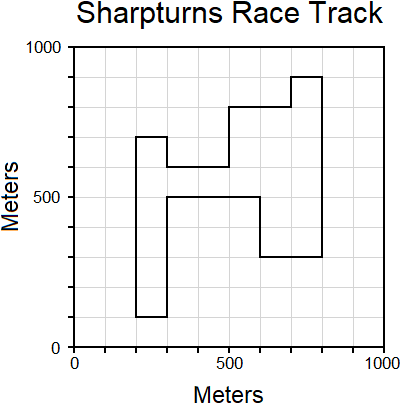### What is the distance traveled when completing one lap around the track?

 A 2900 meters B 4700 meters C 2200 meters D 2000 meters E 3400 meters
Question 40 Explanation:
The correct answer is (E). Each grid space travelled is 100m. The distance travelled in one lap is simply the sum of the grid spaces required to make one lap of the circuit.As you count around the race track you should end up at 34. Giving a total distance of 34 × 100m = 3400m.
 Question 41

### Use the information below to answer the question that follows.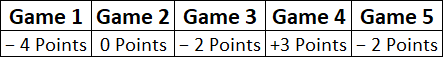### This chart shows the net points scored in five different games. What is the difference between the highest net points scored and the average (mean) net points scored for the 5 games?

 A −3 B 4 C −1 D 2 E 1
Question 41 Explanation:
The correct answer is (B). The highest net points scored occured in Game 4 and was +3 points.

To find the average (mean) points scored for the 5 games we need to find the sum of the points scored and divide by 5 (the number of values being summed):

$\frac{(−4) + (0) +(−2) + (3) + (−2)}{5}$ $=\frac{−5}{5} = −1$

The difference between the highest score and the average score =

(3) − (−1) = 3 + 1 = 4
 Question 42

### What is the ones digit in the divisor of the problem below?A 7 B 3 C 6 D 9 E 5
Question 42 Explanation:

Dividend = Divisor ∗ Quotient + Remainder

150 = $x$ ∗ 8 + 14

We can either guess and check using each answer or use algebra to solve for $x$:
150 = $x$ ∗ 8 + 14
150 − 14 = $x$ ∗ 8
136 = $x$ ∗ 8
$\frac{136}{8} = x$
$\, x$ = 17

The missing ones digit in the divisor = 7
 Question 43

### Alexis has two boards. The first board is $8\frac{11}{16}$ feet long and the second is $11\frac{1}{4}$ feet long. How much longer is the second board than the first?

 A $2 \frac{11}{16}$ feet B $3 \frac{7}{8}$ feet C $3 \frac{5}{16}$ feet D $2 \frac{9}{16}$ feet E $2 \frac{3}{4}$ feet
Question 43 Explanation:
The correct answer is (D). To find the difference in length between the two boards, we need to subtract the shorter length ($8\frac{11}{16}$) from the longer length ($11\frac{1}{4}$).

When dealing with subtracting fractions you must first find a common denominator (the least common multiple of the two denominators). In this case we have denominators of 16 and 4, and the least common multiple is 16.

Since $8\frac{11}{16}$ already has a denominator of 16, no change is required. On the other hand, we need to change $11\frac{1}{4}$ to $11\frac{4}{16}$.

At this point we need to solve: $11\frac{4}{16} − 8\frac{11}{16}$.

The easiest way will be to “count up” from the lower number. To get from $8\frac{11}{16}$ to 9, we need to add $\frac{5}{16}$; to get from 9 to 11 we need to add 2; and to get from 11 to $11\frac{4}{16}$ we need to add $\frac{4}{16}$.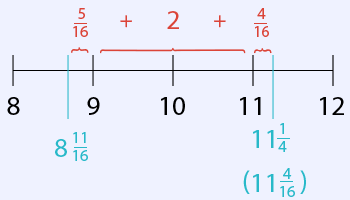$\frac{5}{16} + 2 + \frac{4}{16} = 2\frac{9}{16}$

The difference in length between the two boards is $2 \frac{9}{16}$ feet.
 Question 44

### Use the chart below to answer the question that follows.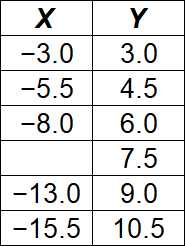### The chart above shows X and Y values from a linear function. Based on the information provided, what is the missing X value?

 A −10.0 B −9.5 C −11.5 D −10.5 E −11.0
Question 44 Explanation:
The correct answer is (D). In a linear function the change in X and Y values will remain constant. We can see that the Y values change by +1.5 (increase by 1.5) each time the X values change by −2.5 (decrease by 2.5).

To determine the missing X value, we need to take −8.0 and subtract (decrease by) 2.5:

−8.0 − 2.5 = −10.5

The missing X value is −10.5.
 Question 45

### Which of the following lists the mixed numbers correctly, creating a true statement?

 A $5\frac{1}{5} \gt 4\frac{4}{5} \gt 4\frac{2}{5}$ B $3\frac{5}{7} \lt 3\frac{2}{7} \lt 4\frac{3}{7}$ C $1\frac{2}{3} \gt 2\frac{1}{3} \gt 1\frac{1}{3}$ D $9\frac{1}{9} \lt 8\frac{8}{9} \lt 8\frac{5}{9}$ E $2\frac{4}{7} \lt 3\frac{1}{7} \lt 2\frac{6}{7}$
Question 45 Explanation:
The correct answer is (A). First it is important to remember the proper orientation of the > (greater than) and < (less than) signs. As silly as it sounds, it might be easiest to picture the symbol as an alligator’s mouth which is always going to “eat” the larger number. In other words, 3 > 2 and 3 < 5. Notice that in both cases the open end of the “mouth” is faced towards the number of greater value.

Next, we need to understand that mixed numbers consist of the number of “wholes” plus a fractional portion. $3\frac{5}{8}$ means “3 wholes plus five-eighths.” You could picture it as 3 whole pizzas plus 5 out of 8 slices of a 4th pizza.

$5\frac{1}{8} \gt 4\frac{7}{8}$. “5 wholes plus 1 slice out of 8” is always going to be more than “4 wholes plus any fraction (in this case 7 slices out of 8).”

Putting all of this together, the only true statement is (A). “5 wholes plus 1 slice out of 5” is greater than “4 wholes plus 4 slices out of 5” which is greater than “4 wholes plus 2 slices out of 5.”
 Question 46

### Which of the following correctly lists three fractions that are each greater than $\frac{1}{2}$?

 A $\dfrac{4}{9}, \dfrac{6}{8}, \dfrac{7}{11}$ B $\dfrac{6}{13}, \dfrac{7}{12}, \dfrac{7}{13}$ C $\dfrac{2}{3}, \dfrac{3}{8}, \dfrac{3}{6}$ D $\dfrac{5}{8}, \dfrac{4}{6}, \dfrac{6}{11}$ E $\dfrac{5}{6}, \dfrac{6}{9}, \dfrac{3}{7}$
Question 46 Explanation:
The correct answer is (D). To determine whether a given fraction is greater than $\frac{1}{2}$, find half the denominator (bottom number); or take the denominator and divide by 2.

If the numerator (top number) is greater than half the denominator then the fraction itself is greater than $\frac{1}{2}$.

In this case: $\frac{5}{8}$ is greater than $\frac{1}{2}$ because 5 is greater than 4 (half of the denominator, 8).

$\frac{4}{6}$is greater than $\frac{1}{2}$ because 4 is greater than 3 (half of the denominator, 6).

$\frac{6}{11}$is greater than $\frac{1}{2}$ because 6 is greater than 5.5 (half of the denominator, 11).
 Question 47

### Yosemite Mule Tours offers three tours of equal length each day. The “45 Minute Break” between tours is used to unload the previous group and prepare the next group of mules. Based on this information and the schedule below, at what time does “Tour Two” end?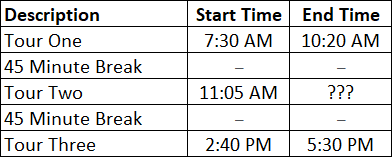A 1:40 PM B 1:55 PM C 3:00 PM D 1:05 PM E 2:10 PM
Question 47 Explanation:
The correct answer is (B). Perhaps the simplest way to approach this question is to recognize that “Tour Two” ends 45 minutes before the beginning of “Tour Three”.

“Tour Three” begins at 2:40 PM. “Tour Two” ends 45 minutes earlier, or 1:55 PM.
 Question 48

### In what year was the difference in the share of steel production between China and North American approximately 25%?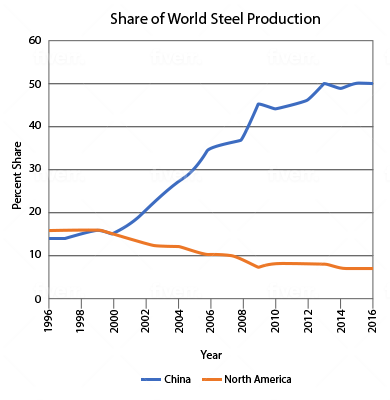A 2006 B 1998 C 2010 D 2014 E 2002
Question 48 Explanation:
The correct answer is (A). In 2008 we see that the Chinese share was approximately 37% (somewhere between 35% and 40%) and the North American share was just below 10%. It is at this point that the difference between China and North America is closest to 25%.
 Question 49

### According to the chart below, in which four-year period listed in the answer choices did United States health care spending as a % of GDP increase the most?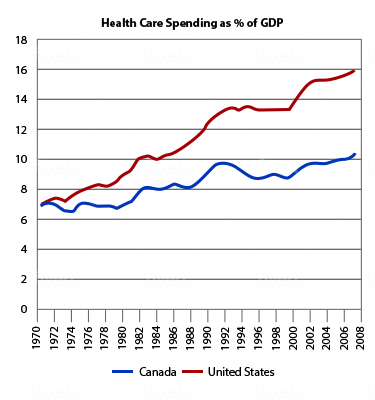A 1972–1976 B 2002–2006 C 1986–1990 D 1982–1986 E 1996–2000
Question 49 Explanation:
The correct answer is (C). From 1986 to 1990 U.S. health care spending as a % of GDP changed from 10.5% to 12.5%, a 2% change. In each of the other four-year periods listed, it changed 1% or less.
 Question 50

### The chart below shows the distance between four different cities. Use this chart to help you answer the question below.### Wes is driving from Cincinnati to Destin. If he has averaged 80 miles per hour so far and has been driving for 2.5 hours, approximately how far is he from Destin?

 A 640 miles B 580 miles C 610 miles D 530 miles E 490 miles
Question 50 Explanation:
The correct answer is (D). Begin by using the chart to determine the total distance from Cincinnati to Destin. It does not matter whether we look in the third column and forth row or the fourth column and third row; in both cases the distance is 730 miles between the cities.

Next, determine the distance traveled in 2.5 hours at an average of 80 mph. If we are traveling 80 miles in one hour, in 2.5 hours we will travel 80 ∗ 2.5 or 80 + 80 + 40 (“all of 80 plus all of 80 plus half of 80”) which gives us 200 miles.

Wes has traveled 200 miles of the 730 miles. This means he has 530 miles (730 − 200) remaining in his trip to Destin.
Once you are finished, click the button below. Any items you have not completed will be marked incorrect.
There are 50 questions to complete.
 ← List →